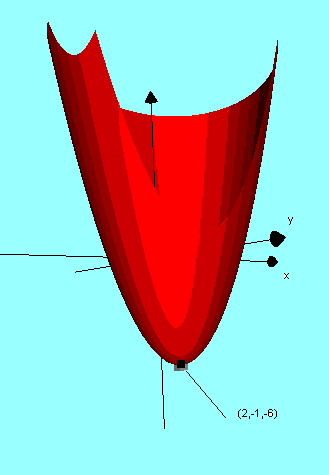# Maxima and Minima of Functions of Two Variables

Locate relative maxima, minima and saddle points of functions of two variables. Several examples with detailed solutions are presented. 3-Dimensional graphs of functions are shown to confirm the existence of these points. More on Optimization Problems with Functions of Two Variables in this web site.

## Theorem

Let f be a function with two variables with continuous second order partial derivatives fxx, fyy and fxy at a critical point (a,b). Let
D = fxx(a,b) fyy(a,b) - fxy2(a,b)
a) If D > 0 and fxx(a,b) > 0, then f has a relative minimum at (a,b).
b) If D > 0 and fxx(a,b) < 0, then f has a relative maximum at (a,b).
c) If D < 0, then f has a saddle point at (a,b).
d) If D = 0, then no conclusion can be drawn.

## Examples with Detailed Solutions

We now present several examples with detailed solutions on how to locate relative minima, maxima and saddle points of functions of two variables. When too many critical points are found, the use of a table is very convenient.

### Example 1

Determine the critical points and locate any relative minima, maxima and saddle points of function f defined by
f(x , y) = 2x2 + 2xy + 2y2 - 6x
.
Solution to Example 1:
Find the first partial derivatives fx and fy.
fx(x,y) = 4x + 2y - 6
fy(x,y) = 2x + 4y
The critical points satisfy the equations fx(x,y) = 0 and fy(x,y) = 0 simultaneously. Hence.
4x + 2y - 6 = 0
2x + 4y = 0
The above system of equations has one solution at the point (2,-1).
We now need to find the second order partial derivatives fxx(x,y), fyy(x,y) and fxy(x,y).
fxx(x,y) = 4
fyy(x,y) = 4
fxy(x,y) = 2
We now need to find D defined above.
D = fxx(2,-1) fyy(2,-1) - fxy2(2,-1) = ( 4 )( 4 ) - 22 = 12
Since D is positive and fxx(2,-1) is also positive, according to the above theorem function f has a local minimum at (2,-1).
The 3-Dimensional graph of function f given above shows that f has a local minimum at the point (2,-1,f(2,-1)) = (2,-1,-6).### Example 2

Determine the critical points and locate any relative minima, maxima and saddle points of function f defined by
f(x , y) = 2x2 - 4xy + y4 + 2
.

Solution to Example 2:
Find the first partial derivatives fx and fy.
fx(x,y) = 4x - 4y
fy(x,y) = - 4x + 4y3
Determine the critical points by solving the equations fx(x,y) = 0 and fy(x,y) = 0 simultaneously. Hence.
4x - 4y = 0
- 4x + 4y3 = 0
The first equation gives x = y. Substitute x by y in the equation - 4x + 4y3 = 0 to obtain.
- 4y + 4y3 = 0
Factor and solve for y.
4y(-1 + y2) = 0
y = 0 , y = 1 and y = -1
We now use the equation x = y to find the critical points.
(0 , 0) , (1 , 1) and (-1 , -1)
We now determine the second order partial derivatives.
fxx(x,y) = 4
fyy(x,y) = 12y2
fxy(x,y) = -4
We now use a table to study the signs of D and fxx(a,b) and use the above theorem to decide on whether a given critical point is a saddle point, relative maximum or minimum.

 critical point (a,b) (0,0) (1,1) (-1,1) fxx(a,b) 4 4 4 fyy(a,b) 0 12 12 fxy(a,b) -4 -4 -4 D -16 32 32 saddle point relative minimum relative minimum

A 3-Dimensional graph of function f shows that f has two local minima at (-1,-1,1) and (1,1,1) and one saddle point at (0,0,2).### Example 3

Determine the critical points and locate any relative minima, maxima and saddle points of function f defined by
f(x , y) = - x4 - y4 + 4xy
.

Solution to Example 3:
First partial derivatives fx and fy are given by.
fx(x,y) = - 4x3 + 4y
fy(x,y) = - 4y3 + 4x
We now solve the equations fy(x,y) = 0 and fx(x,y) = 0 to find the critical points..
- 4x3 + 4y = 0
- 4y3 + 4x = 0
The first equation gives y = x3. Combined with the second equation, we obtain.
- 4(x3)3 + 4x = 0
Which may be written as .
x(x4 - 1)(x4 + 1) = 0
Which has the solutions.
x = 0 , -1 and 1.
We now use the equation y = x3 to find the critical points.
(0 , 0) , (1 , 1) and (-1 , -1)
We now determine the second order partial derivatives.
fxx(x,y) = -12x2
fyy(x,y) = -12y2
fxy(x,y) = 4
The table below shows the signs of D and fxx(a,b). Then the above theorem is used to decide on what type of critical points it is.

 critical point (a,b) (0,0) (1,1) (-1,1) fxx(a,b) 0 -12 -12 fyy(a,b) 0 -12 -12 fxy(a,b) 4 4 4 D -16 128 128 saddle point relative maximum relative maximum

A 3-Dimensional graph of function f shows that f has two local maxima at (-1,-1,2) and (1,1,2) and a saddle point at (0,0,0).## Exercises

Determine the critical points of the functions below and find out whether each point corresponds to a relative minimum, maximum, saddle point or no conclusion can be made.
1. f(x , y) = x2 + 3 y2 - 2 xy - 8x
2. f(x , y) = x3 - 12 x + y3 + 3 y2 - 9y

## Answers to the Above Exercises

1. relative maximum at (1,1) and (-1,-1) and a saddle point at (0,0)
2. relative maximum at (2,-3), relative minimum at (2,1), saddle points at (-2,-3) and (-2,1).

## More Links on Partial Derivatives and Multivariable Functions

Multivariable Functions